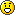Forum » Maths Problem

## Maths ProblemNarvius 12 years ago sqrt(a) + sqrt(b) = sqrt(4 + sqrt(7))Find all real solutions (a, b).I have no idea, and I somehow doubt thatfor all 0 < sqrt(a) < sqrt(4 + sqrt(7)) :: sqrt(b) = sqrt(4 + sqrt(7)) - sqrt(a) therefore for all 0 < a < 4 + sqrt(7) :: b = (sqrt(4 + sqrt(7)) - sqrt(a)) ^ 2is a sufficient answer.Halp. # quoteAmarth 12 years ago Sounds right to me?You can expand the last equation tob = 4 + sqrt(7) - 2 * sqrt( (4 + sqrt(7)) * a ) + abut that's about all you can say about it I guess. # quoteNarvius 12 years ago Okay then... it just seems too easy to me.The more fun one:Prove that for any array P containing 3^k or more integers, it's possible to pick a subarray S containing (k + 1) items, in a way that for no two (different) subarrays of S the sum of their items are the same. # quoteE_net4 12 years ago Well, you're dealing with subarrays of a subarray there. Have you tried through mathematical induction? Though it's strange that the sum of both subarrays will always be the same if all array items are zero. # quoteAmarth 12 years ago I'm going to guess it's not about arrays but about sets, ie no repetition of integers.This problem sounds like mathematical induction and/or the pigeonhole principle. Hmm. I don't immediately see it and I don't have much time to think about it right now, but maybe later. # quoteE_net4 12 years ago "Amarth" said:I'm going to guess it's not about arrays but about sets, ie no repetition of integers.Now that really changes the whole thing.Let's assume that.For k = 0, we have a subset of k + 1 = 1 elements. Then we can make an empty subset of this one and another one with this single item... But the sum of both could STILL be 0. Narvius, you'll have to be more specific. # quote
Forum » Maths ProblemYour email: Your name: